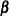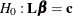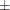### TEST Statement

<label:> TEST equation1 <,, <equationk>> </ options> ;

The TEST statement tests linear hypotheses about the parameters. An F test is used to jointly test the null hypotheses () specified in a single TEST statement in which the MULT option is specified.

Each equation specifies a linear hypothesis (a row of thematrix and the corresponding element of thevector); multiple equations are separated by commas. The label, which must be a valid SAS name, is used to identify the resulting output. You can submit multiple TEST statements. When a label is not included in a TEST statement, a label of Test j is used for the jth TEST statement.

The form of an equation is as follows:

term <term>   < =term <term> >

where term is a parameter of the model, or a constant, or a constant times a parameter. When no equal sign appears, the expression is set to 0. Only parameters for regressor effects (continuous variables by themselves) are allowed.

For each TEST statement, PROC MIANALYZE displays a Test Specification table of thematrix and thevector. The procedure also displays a Variance Information table of the between-imputation, within-imputation, and total variances for combining complete-data inferences, and a Parameter Estimates table of a combined estimate and standard error for each linear component. The linear components are labeled TestPrm1, TestPrm2, ... in the tables.

The following statements illustrate possible uses of the TEST statement:

proc mianalyze;
modeleffects intercept a1 a2 a3;
test1: test intercept + a2 = 0;
test2: test intercept + a2;
test3: test a1=a2=a3;
test4: test a1=a2, a2=a3;
run;


The first and second TEST statements are equivalent and correspond to the specification in Figure 58.5.

Figure 58.5: Test Specification for test1 and test2

The MIANALYZE Procedure
Test: test1

Test Specification
Parameter L Matrix C
intercept a1 a2 a3
TestPrm1 1.000000 0 1.000000 0 0

The third and fourth TEST statements are also equivalent and correspond to the specification in Figure 58.6.

Figure 58.6: Test Specification for test3 and test4

The MIANALYZE Procedure
Test: test3

Test Specification
Parameter L Matrix C
intercept a1 a2 a3
TestPrm1 0 1.000000 -1.000000 0 0
TestPrm2 0 0 1.000000 -1.000000 0

The ALPHA= and EDF options specified in the PROC MIANALYZE statement are also applied to the TEST statement. You can specify the following options in the TEST statement after a slash(/):

BCOV

displays the between-imputation covariance matrix.

MULT

displays the multivariate inference for parameters.

TCOV

displays the total covariance matrix.

WCOV

displays the within-imputation covariance matrix.